#### Cylindrical decomposition

The cylindrical decomposition is very similar to the vertical decomposition, except that when any of the cases in Figure 6.2 occurs, then a vertical line slices through all faces, all the way from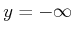to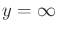. The result is shown in Figure 6.18, which may be considered as a singular complex. This may appear very inefficient in comparison to the vertical decomposition; however, it is presented here because it generalizes nicely to any dimension, any C-space topology, and any semi-algebraic model. Therefore, it is presented here to ease the transition to more general decompositions. The most important property of the cylindrical decomposition is shown in Figure 6.19. Consider each vertical strip between two events. When traversing a strip fromto, the points alternate between being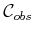and. For example, between events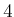and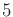, the points below edge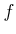are in. Points betweenand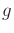lie in. Points betweenand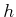lie in, and so forth. The cell decomposition can be defined so that 2D cells are also created in. Let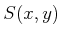denote the logical predicate (3.6) from Section 3.1.1. When traversing a strip, the value ofalso alternates. This behavior is the main reason to construct a cylindrical decomposition, which will become clearer in Section 6.4.2. Each vertical strip is actually considered to be a cylinder, hence, the name cylindrical decomposition (i.e., there are not necessarily any cylinders in the 3D geometric sense).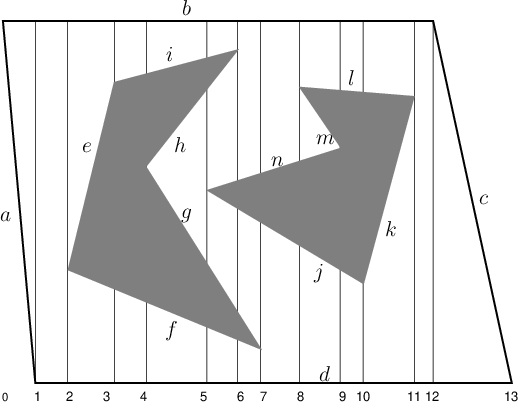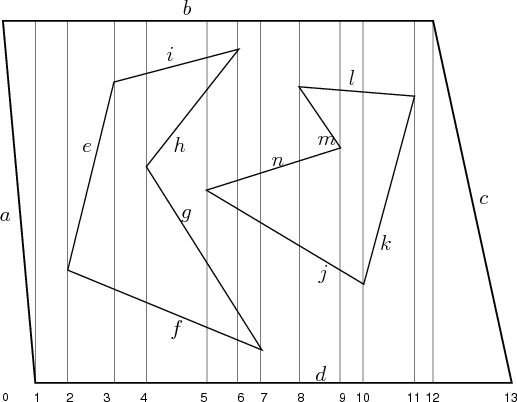Steven M LaValle 2020-08-14